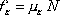f-sub-K EQUALS mu-sub-K TIMES N

The force of kinetic friction is equal to the coefficient of kinetic friction times the normal force.

Kinetic friction, also known as sliding friction, is the frictional force exerted by a surface upon an object that is sliding along that surface.

Pitfall Avoidance Note: mu-sub-K is not the frictional force. mu-sub-K is the coefficient of kinetic friction. The value of mu-sub-K can only be a pure number, a value with no units. The force of kinetic friction does depend on mu-sub-K, but it is never equal to mu-sub-K. It can't be. The quantity mu-sub-K does not even have units of force.